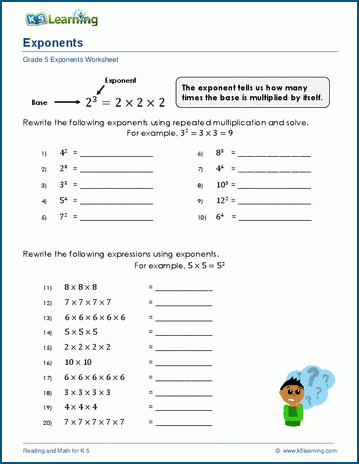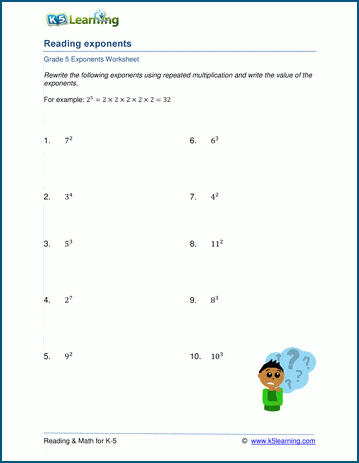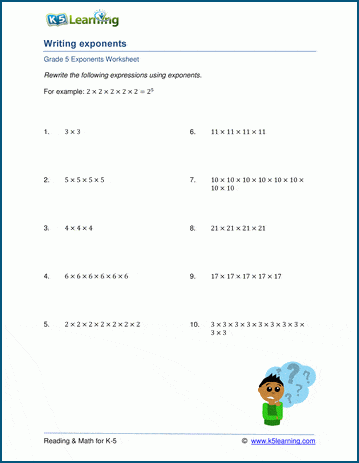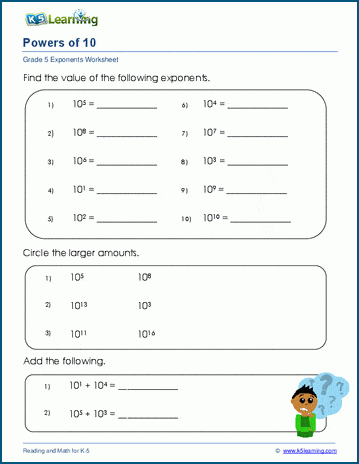The K5 Learning Blog urges parents to be pro-active in helping their children reach their full academic potential.

K5 Learning
provides an online reading and math program for kindergarten to grade 5 students.In grade 5, students start learning about exponent math and about the properties of exponents.  We created a new page of exponent worksheets in our grade 5 math section that covers introducing, reading and writing exponents.

Introducing exponents

This set of worksheets introduces exponents: the repeated multiplication of a base number, and shows students how to rewrite a number being multiplied by itself as an exponent.Reading exponents

The next step is to give the students simple practice of exponents.  In these worksheets, where all base numbers are less than 15, students are given a simple expression with exponents and are asked to calculate the answer.Writing exponents

In these worksheets, students are asked to rewrite the expression as an exponent.Powers of ten

Powers of ten is a useful way of writing down very large number or very small numbers. Instead of showing lots of zeros, you show how many powers of ten make that many zeros. For example, 103 is 1,000. This page contains worksheets that get incrementally harder, starting with evaluating simple power of ten, to rewriting large numbers as powers of ten.